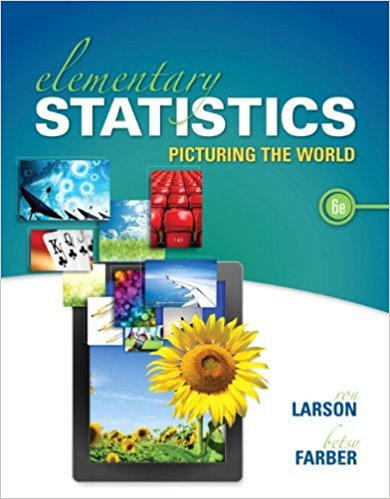×
×

# Solutions for Chapter 10: CHI-SQUARE TESTS AND THE F-DISTRIBUTION## Full solutions for Elementary Statistics: Picturing the World | 6th Edition

ISBN: 9780321911216Solutions for Chapter 10: CHI-SQUARE TESTS AND THE F-DISTRIBUTION

Solutions for Chapter 10
4 5 0 240 Reviews
25
2
##### ISBN: 9780321911216

Elementary Statistics: Picturing the World was written by and is associated to the ISBN: 9780321911216. Chapter 10: CHI-SQUARE TESTS AND THE F-DISTRIBUTION includes 22 full step-by-step solutions. Since 22 problems in chapter 10: CHI-SQUARE TESTS AND THE F-DISTRIBUTION have been answered, more than 118834 students have viewed full step-by-step solutions from this chapter. This textbook survival guide was created for the textbook: Elementary Statistics: Picturing the World , edition: 6. This expansive textbook survival guide covers the following chapters and their solutions.

Key Statistics Terms and definitions covered in this textbook
• Attribute control chart

Any control chart for a discrete random variable. See Variables control chart.

• Bayes’ estimator

An estimator for a parameter obtained from a Bayesian method that uses a prior distribution for the parameter along with the conditional distribution of the data given the parameter to obtain the posterior distribution of the parameter. The estimator is obtained from the posterior distribution.

• Chance cause

The portion of the variability in a set of observations that is due to only random forces and which cannot be traced to speciic sources, such as operators, materials, or equipment. Also called a common cause.

• Completely randomized design (or experiment)

A type of experimental design in which the treatments or design factors are assigned to the experimental units in a random manner. In designed experiments, a completely randomized design results from running all of the treatment combinations in random order.

• Conditional probability density function

The probability density function of the conditional probability distribution of a continuous random variable.

• Conditional variance.

The variance of the conditional probability distribution of a random variable.

• Consistent estimator

An estimator that converges in probability to the true value of the estimated parameter as the sample size increases.

• Contrast

A linear function of treatment means with coeficients that total zero. A contrast is a summary of treatment means that is of interest in an experiment.

• Correlation matrix

A square matrix that contains the correlations among a set of random variables, say, XX X 1 2 k , ,…, . The main diagonal elements of the matrix are unity and the off-diagonal elements rij are the correlations between Xi and Xj .

• Cumulative normal distribution function

The cumulative distribution of the standard normal distribution, often denoted as ?( ) x and tabulated in Appendix Table II.

• Deming

W. Edwards Deming (1900–1993) was a leader in the use of statistical quality control.

• Density function

Another name for a probability density function

• Discrete distribution

A probability distribution for a discrete random variable

• Discrete uniform random variable

A discrete random variable with a inite range and constant probability mass function.

• Distribution free method(s)

Any method of inference (hypothesis testing or conidence interval construction) that does not depend on the form of the underlying distribution of the observations. Sometimes called nonparametric method(s).

• Error of estimation

The difference between an estimated value and the true value.

• Estimator (or point estimator)

A procedure for producing an estimate of a parameter of interest. An estimator is usually a function of only sample data values, and when these data values are available, it results in an estimate of the parameter of interest.

• Event

A subset of a sample space.

• F-test

Any test of signiicance involving the F distribution. The most common F-tests are (1) testing hypotheses about the variances or standard deviations of two independent normal distributions, (2) testing hypotheses about treatment means or variance components in the analysis of variance, and (3) testing signiicance of regression or tests on subsets of parameters in a regression model.

• Generator

Effects in a fractional factorial experiment that are used to construct the experimental tests used in the experiment. The generators also deine the aliases.

×